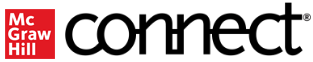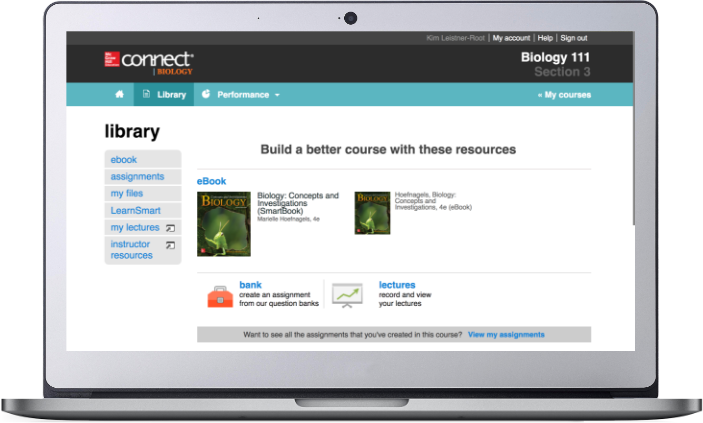## Services

#### Support# Precalculus, 1st Edition

ISBN10: 0078035600 | ISBN13: 9780078035609
By Julie Miller, Donna Gerken

## Purchase Options

* The estimated amount of time this product will be on the market is based on a number of factors, including faculty input to instructional design and the prior revision cycle and updates to academic research-which typically results in a revision cycle ranging from every two to four years for this product. Pricing subject to change at any time.

## Program Details

Julie Miller wrote her developmental math series because students were coming into her Precalculus course underprepared. They weren’t mathematically mature enough to understand the concepts of math nor were they fully engaged with the material. She began her developmental mathematics offerings with intermediate algebra to help bridge that gap. The Precalculus series is a carefully constructed end to that bridge that uses the highly effective pedagogical features from her fastest growing developmental math series. What sets Julie Miller’s series apart is that it addresses course issues through an author-created digital package that maintains a consistent voice and notation throughout the program. This consistency--in videos, PowerPoints, Lecture Notes, and Group Activities--coupled with the power of ALEKS and Connect Hosted by ALEKS, ensures that students master the skills necessary to be successful in Precalculus and can carry them through to the calculus sequence.

### Chapter R: Review of Prerequisites

Section R.1 Sets and the Real Number Line Section R.2 Exponents and Radicals Section R.3 Polynomials and Factoring Obj 8 Factor Expressions Containing Negative and Rational Exponents Problem Recognition Exercises Simplifying Algebraic Expressions Section R.4 Rational Expressions and More Operations on Radicals Section R.5 Equations Section R.6 Complex Numbers and Equations with Complex Solutions Section R.7 Linear, Compound, and Absolute Value Inequalities Section R.8 Applications of Equations and Inequalities

### Chapter 1: Functions and Relations

Section 1.1 The Rectangular Coordinate System and Graphing Utilities Section 1.2 Circles Section 1.3 Functions and Relations Section 1.4 Linear Equations in Two Variables and Linear Functions Section 1.5 Applications of Linear Equations and Modeling Problem Recognition Exercises Comparing Graphs of Equations Section 1.6 Transformations of Graphs Section 1.7 Analyzing Graphs of Functions and Piecewise-Defined Functions Section 1.8 Algebra of Functions and Function Composition

### Chapter 2: Polynomial and Rational Functions

Section 2.1 Quadratic Functions and Applications Section 2.2 Introduction to Polynomial Functions Section 2.3 Division of Polynomials and the Remainder and Factor Theorems Section 2.4 Zeros of Polynomials Section 2.5 Rational Functions Problem Recognition Exercises Polynomial and Rational Functions Section 2.6 Polynomial and Rational Inequalities Problem Recognition Exercises Solving Equations and Inequalities Section 2.7 Variation

### Chapter 3: Exponential and Logarithmic Functions

Section 3.1 Inverse Functions Section 3.2 Exponential Functions Section 3.3 Logarithmic Functions Problem Recognition Exercises Analyzing Functions Section 3.4 Properties of Logarithms Section 3.5 Exponential and Logarithmic Equations Section 3.6 Modeling with Exponential and Logarithmic Functions

### Chapter 4: Trigonometric Functions

Section 4.1 Angles and Their Measure and Special Triangles Section 4.2 Trigonometric Functions Defined on the Unit Circle Section 4.3 Trigonometric Functions Defined on Right Triangles Section 4.4 Graphs of the Sine and Cosine Functions Section 4.5 Graphs of Other Trigonometric Functions Problem Recognition Exercises Comparing Graphical Characteristics of Trigonometric Functions Section 4.6 Inverse Trigonometric Functions

### Chapter 5: Analytic Trigonometry

Section 5.1 Trigonometric Identities Section 5.2 Sum and Difference Formulas Section 5.3 Double-Angle and Half-Angle Formulas Section 5.4 Product-to-Sum and Sum-to-Product Formulas Section 5.5 Trigonometric Equations

### Chapter 6: Applications of Trigonometric Functions

Section 6.1 Applications of Right Triangles Section 6.2 The Law of Sines Section 6.3 The Law of Cosines Problem Recognition Exercises Solving Triangles Using a Variety of Tools Section 6.4 Harmonic Motion and Combinations of Trigonometric Functions

### Chapter 7: Trigonometry Applied to Rectangular and Polar Coordinate Systems and Vectors

Section 7.1 Polar Coordinates Section 7.2 Graphs of Polar Equations Problem Recognition Exercises Comparing Equations in Polar and Rectangular Form Section 7.3 Complex Numbers in Polar Form Section 7.4 Vectors Section 7.5 Dot Product

### Chapter 8: Systems of Equations and Inequalities

Section 8.1 Systems of Linear Equations in Two Variables and Applications Section 8.2 Systems of Linear Equations in Three Variables and Applications Section 8.3 Partial Fraction Decomposition Section 8.4 Systems of Nonlinear Equations in Two Variables Section 8.5 Inequalities and Systems of Inequalities in Two Variables Problem Recognition Exercises Equations and Inequalities in Two Variables Section 8.6 Linear Programming

### Chapter 9: Matrices and Determinants and Applications

Section 9.1 Solving Systems of Linear Equations Using Matrices Section 9.2 Inconsistent Systems and Dependent Equations Section 9.3 Operations on Matrices Section 9.4 Inverse Matrices and Matrix Equations Section 9.5 Determinants and Cramer's Rule Problem Recognition Exercises Using Multiple Methods to Solve Systems of Linear Equations

### Chapter 10: Analytic Geometry

Section 10.1 The Ellipse Section 10.2 The Hyperbola Section 10.3 The Parabola Problem Recognition Exercises Comparing Equations of Conic Sections and the General Equation Section 10.4 Rotation of Axes Section 10.5 Polar Equations of Conics Section 10.6 Plane Curves and Parametric Equations

### Chapter 11: Sequences, Series, Induction, and Probability

Section 11.1 Sequences and Series Section 11.2 Arithmetic Sequences and Series Section 11.3 Geometric Sequences and Series Problem Recognition Exercises Comparing Arithmetic and Geometric Sequences and Series Section 11.4 Mathematical Induction Section 11.5 The Binomial Theorem Section 11.6 Principles of Counting Section 11.7 Introduction to Probability

### Online: Chapter 12: Preview of Calculus

Section 12.1 Introduction to Limits through Tables and Graphs Section 12.2 Algebraic Properties of Limits Problem Recognition Exercises Using a Variety of Methods to Evaluate Limits Section 12.3 Derivatives: The Tangent Problem Section 12.4 Integrals: The Area Problem

#### Online: Section A-1 Proof of the Binomial Theorem A-2 Definition of Conics From a Fixed Point and Fixed LineBy prompting students to engage with key concepts, while continually adapting to their individual needs, Connect activates learning and empowers students to take control resulting in better grades and increased retention rates. Proven online content integrates seamlessly with our adaptive technology, and helps build student confidence outside of the classroom.

#### SmartBook® 2.0

Available within Connect, SmartBook 2.0 is an adaptive learning solution that provides personalized learning to individual student needs, continually adapting to pinpoint knowledge gaps and focus learning on concepts requiring additional study. SmartBook 2.0 fosters more productive learning, taking the guesswork out of what to study, and helps students better prepare for class. With the ReadAnywhere mobile app, students can now read and complete SmartBook 2.0 assignments both online and off-line. For instructors, SmartBook 2.0 provides more granular control over assignments with content selection now available at the concept level. SmartBook 2.0 also includes advanced reporting features that enable instructors to track student progress with actionable insights that guide teaching strategies and advanced instruction, for a more dynamic class experience.Your text has great instructor tools, like presentation slides, instructor manuals, test banks and more. Follow the steps below to access your instructor resources or watch the step-by-step video.

1. To get started, visit connect.mheducation.com to sign in. (If you do not have an account, request one from your McGraw Hill rep. To find your rep, visit Find Your Rep)
2. Then, under "Find a Title," search by title, author, or subject
3. Select your desired title, and create a course. (You do not have to create assignments, just a course instance)
4. Go to your Connect course homepage
5. In the top navigation, select library to access the title's instructor resources